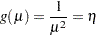Distribution Family and Canonical Link

In general, there is not just one reasonable link function for a given response variable distribution. For parametric models, the choice of link function can lead to substantively different estimates and tests. However, the inherent flexibility of nonparametric models makes them less likely to be sensitive to the precise choice of link function. Thus, for simplicity and computational efficiency, the GAM procedure uses only the canonical link for each distribution, as discussed in the following sections.

### The Gaussian Model

For a Gaussian model, the link function is the identity function, and the generalized additive model is the additive model. The Gaussian model is selected by default or when you specify the DIST=GAUSSIAN option in the MODEL statement.

### The Binomial Model

The binomial model is selected by specifying the DIST=BINOMIAL option in the MODEL statement. A binomial response model assumes that the proportion of successesis such thathas a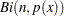distribution.refers to the binomial distribution with the parametersand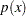. Often the data are binary, in which case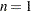. The canonical link is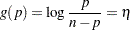By default, PROC GAM models the probability of the response level with the lower ordered value. Ordered values are assigned to response levels in ascending sorted order and are displayed in the “Response Profiles” table. For binary data, if your event category has a higher Ordered Value, then by default the nonevent is modeled. The effect of modeling the nonevent is to change the signs of the estimated coefficients for linear terms in the model for the event. You can change which probability is modeled by specifying the EVENT=, DESCENDING, or ORDER= response variable options in the MODEL statement.

### The Poisson Model

The Poisson model is selected by specifying the DIST=POISSON option in the MODEL statement. The link function for the Poisson model is the log function. Assuming that the mean of the Poisson distribution is, the dependence ofand independent variables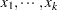is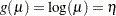### The Gamma Model

The gamma model is selected by specifying the DIST=GAMMA option in the MODEL statement. Let the mean of the gamma distribution be. The canonical link function for the gamma distribution is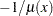. Note that this link function is the negative of the default link function in PROC GENMOD for a gamma model. The relationship betweenand the independent variablesis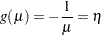### The Inverse Gaussian Model

The inverse Gaussian model is selected by specifying the DIST=IGAUSSIAN option in the MODEL statement. Let the mean of the inverse Gaussian distribution be. The canonical link function for inverse Gaussian distribution is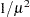. Therefore, the relationship betweenand the independent variablesis